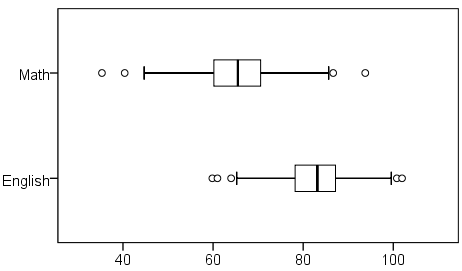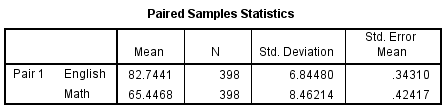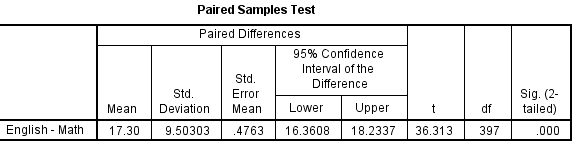# SPSS Tutorials: Paired Samples t Test

Paired t tests are used to test if the means of two paired measurements, such as pretest/posttest scores, are significantly different.

## Paired Samples t Test

The Paired Samples t Test compares the means of two measurements taken from the same individual, object, or related units. These "paired" measurements can represent things like:

• A measurement taken at two different times (e.g., pre-test and post-test score with an intervention administered between the two time points)
• A measurement taken under two different conditions (e.g., completing a test under a "control" condition and an "experimental" condition)
• Measurements taken from two halves or sides of a subject or experimental unit (e.g., measuring hearing loss in a subject's left and right ears).

The purpose of the test is to determine whether there is statistical evidence that the mean difference between paired observations is significantly different from zero. The Paired Samples t Test is a parametric test.

This test is also known as:

• Dependent t Test
• Paired t Test
• Repeated Measures t Test

The variable used in this test is known as:

• Dependent variable, or test variable (continuous), measured at two different times or for two related conditions or units

## Common Uses

The Paired Samples t Test is commonly used to test the following:

• Statistical difference between two time points
• Statistical difference between two conditions
• Statistical difference between two measurements
• Statistical difference between a matched pair

Note: The Paired Samples t Test can only compare the means for two (and only two) related (paired) units on a continuous outcome that is normally distributed. The Paired Samples t Test is not appropriate for analyses involving the following: 1) unpaired data; 2) comparisons between more than two units/groups; 3) a continuous outcome that is not normally distributed; and 4) an ordinal/ranked outcome.

• To compare unpaired means between two independent groups on a continuous outcome that is normally distributed, choose the Independent Samples t Test.
• To compare unpaired means between more than two groups on a continuous outcome that is normally distributed, choose ANOVA.
• To compare paired means for continuous data that are not normally distributed, choose the nonparametric Wilcoxon Signed-Ranks Test.
• To compare paired means for ranked data, choose the nonparametric Wilcoxon Signed-Ranks Test.

## Data Requirements

Your data must meet the following requirements:

1. Dependent variable that is continuous (i.e., interval or ratio level)
1. Note: The paired measurements must be recorded in two separate variables.
2. Related samples/groups (i.e., dependent observations)
1. The subjects in each sample, or group, are the same. This means that the subjects in the first group are also in the second group.
3. Random sample of data from the population
4. Normal distribution (approximately) of the difference between the paired values
5. No outliers in the difference between the two related groups

Note: When testing assumptions related to normality and outliers, you must use a variable that represents the difference between the paired values - not the original variables themselves.

Note: When one or more of the assumptions for the Paired Samples t Test are not met, you may want to run the nonparametric Wilcoxon Signed-Ranks Test instead.

## Hypotheses

The hypotheses can be expressed in two different ways that express the same idea and are mathematically equivalent:

H0: µ1 = µ2 ("the paired population means are equal")
H1: µ1 ≠ µ2 ("the paired population means are not equal")

OR

H0: µ1 - µ2 = 0 ("the difference between the paired population means is equal to 0")
H1µ1 - µ2 ≠ 0 ("the difference between the paired population means is not 0")

where

• µ1 is the population mean of variable 1, and
• µ2 is the population mean of variable 2.

## Test Statistic

The test statistic for the Paired Samples t Test, denoted t, follows the same formula as the one sample t test.

$$t = \frac{\overline{x}_{\mathrm{diff}}-0}{s_{\overline{x}}}$$

where

$$s_{\overline{x}} = \frac{s_{\mathrm{diff}}}{\sqrt{n}}$$

where

$$\bar{x}_{\mathrm{diff}}$$ = Sample mean of the differences
$$n$$ = Sample size (i.e., number of observations)
$$s_{\mathrm{diff}}$$= Sample standard deviation of the differences
$$s_{\bar{x}}$$ = Estimated standard error of the mean (s/sqrt(n))

The calculated t value is then compared to the critical t value with df = n - 1 from the t distribution table for a chosen confidence level. If the calculated t value is greater than the critical t value, then we reject the null hypothesis (and conclude that the means are significantly different).

## Data Set-Up

Your data should include two continuous numeric variables (represented in columns) that will be used in the analysis. The two variables should represent the paired variables for each subject (row). If your data are arranged differently (e.g., cases represent repeated units/subjects), simply restructure the data to reflect this format.

## Run a Paired Samples t Test

To run a Paired Samples t Test in SPSS, click Analyze > Compare Means > Paired-Samples T Test.The Paired-Samples T Test window opens where you will specify the variables to be used in the analysis. All of the variables in your dataset appear in the list on the left side. Move variables to the right by selecting them in the list and clicking the blue arrow buttons. You will specify the paired variables in the Paired Variables area.A Pair: The “Pair” column represents the number of Paired Samples t Tests to run. You may choose to run multiple Paired Samples t Tests simultaneously by selecting multiple sets of matched variables. Each new pair will appear on a new line.

B Variable1: The first variable, representing the first group of matched values. Move the variable that represents the first group to the right where it will be listed beneath the “Variable1 column.

C Variable2: The second variable, representing the second group of matched values. Move the variable that represents the second group to the right where it will be listed beneath the “Variable2” column.

D Options: Clicking Options will open a window where you can specify the Confidence Interval Percentage and how the analysis will address Missing Values (i.e., Exclude cases analysis by analysis or Exclude cases listwise). Click Continue when you are finished making specifications.• Setting the confidence interval percentage does not have any impact on the calculation of the p-value.
• If you are only running one paired samples t test, the two "missing values" settings will produce the same results. There will only be differences if you are running 2 or more paired samples t tests. (This would look like having two or more rows in the main Paired Samples T Test dialog window.)

## Example

### Problem Statement

The sample dataset has placement test scores (out of 100 points) for four subject areas: English, Reading, Math, and Writing. Students in the sample completed all 4 placement tests when they enrolled in the university. Suppose we are particularly interested in the English and Math sections, and want to determine whether students tended to score higher on their English or Math test, on average. We could use a paired t test to test if there was a significant difference in the average of the two tests.

### Before the Test

Variable English has a high of 101.95 and a low of 59.83, while variable Math has a high of 93.78 and a low of 35.32 (Analyze > Descriptive Statistics > Descriptives). The mean English score is much higher than the mean Math score (82.79 versus 65.47). Additionally, there were 409 cases with non-missing English scores, and 422 cases with non-missing Math scores, but only 398 cases with non-missing observations for both variables. (Recall that the sample dataset has 435 cases in all.)

Let's create a comparative boxplot of these variables to help visualize these numbers. Click Analyze > Descriptive Statistics > Explore. Add English and Math to the Dependents box; then, change the Display option to Plots. We'll also need to tell SPSS to put these two variables on the same chart. Click the Plots button, and in the Boxplots area, change the selection to Dependents Together. You can also uncheck Stem-and-leaf. Click Continue. Then click OK to run the procedure.We can see from the boxplot that the center of the English scores is much higher than the center of the Math scores, and that there is slightly more spread in the Math scores than in the English scores. Both variables appear to be symmetrically distributed. It's quite possible that the paired samples t test could come back significant.

### Running the Test

1. Click Analyze > Compare Means > Paired-Samples T Test.
2. Select the variable English and move it to the Variable1 slot in the Paired Variables box. Then select the variable Math and move it to the Variable2 slot in the Paired Variables box.
3. Click OK.

#### Syntax

T-TEST PAIRS=English WITH Math (PAIRED)
/CRITERIA=CI(.9500)
/MISSING=ANALYSIS.

### Output

#### Tables

There are three tables: Paired Samples Statistics, Paired Samples Correlations, and Paired Samples Test. Paired Samples Statistics gives univariate descriptive statistics (mean, sample size, standard deviation, and standard error) for each variable entered. Notice that the sample size here is 398; this is because the paired t-test can only use cases that have non-missing values for both variables. Paired Samples Correlations shows the bivariate Pearson correlation coefficient (with a two-tailed test of significance) for each pair of variables entered. Paired Samples Test gives the hypothesis test results.The Paired Samples Statistics output repeats what we examined before we ran the test. The Paired Samples Correlation table adds the information that English and Math scores are significantly positively correlated (r = .243).

Why does SPSS report the correlation between the two variables when you run a Paired t Test? Although our primary interest when we run a Paired t Test is finding out if the means of the two variables are significantly different, it's also important to consider how strongly the two variables are associated with one another, especially when the variables being compared are pre-test/post-test measures. For more information about correlation, check out the Pearson Correlation tutorial.• First column: The pair of variables being tested, and the order the subtraction was carried out. (If you have specified more than one variable pair, this table will have multiple rows.)
• Mean: The average difference between the two variables.
• Standard deviation: The standard deviation of the difference scores.
• Standard error mean: The standard error (standard deviation divided by the square root of the sample size). Used in computing both the test statistic and the upper and lower bounds of the confidence interval.
• t: The test statistic (denoted t) for the paired T test.
• df: The degrees of freedom for this test.
• Sig. (2-tailed): The p-value corresponding to the given test statistic t with degrees of freedom df.

### Decision and Conclusions

From the results, we can say that:

• English and Math scores were weakly and positively correlated (r = 0.243, p < 0.001).
• There was a significant average difference between English and Math scores (t397 = 36.313, p < 0.001).
• On average, English scores were 17.3 points higher than Math scores (95% CI [16.36, 18.23]).